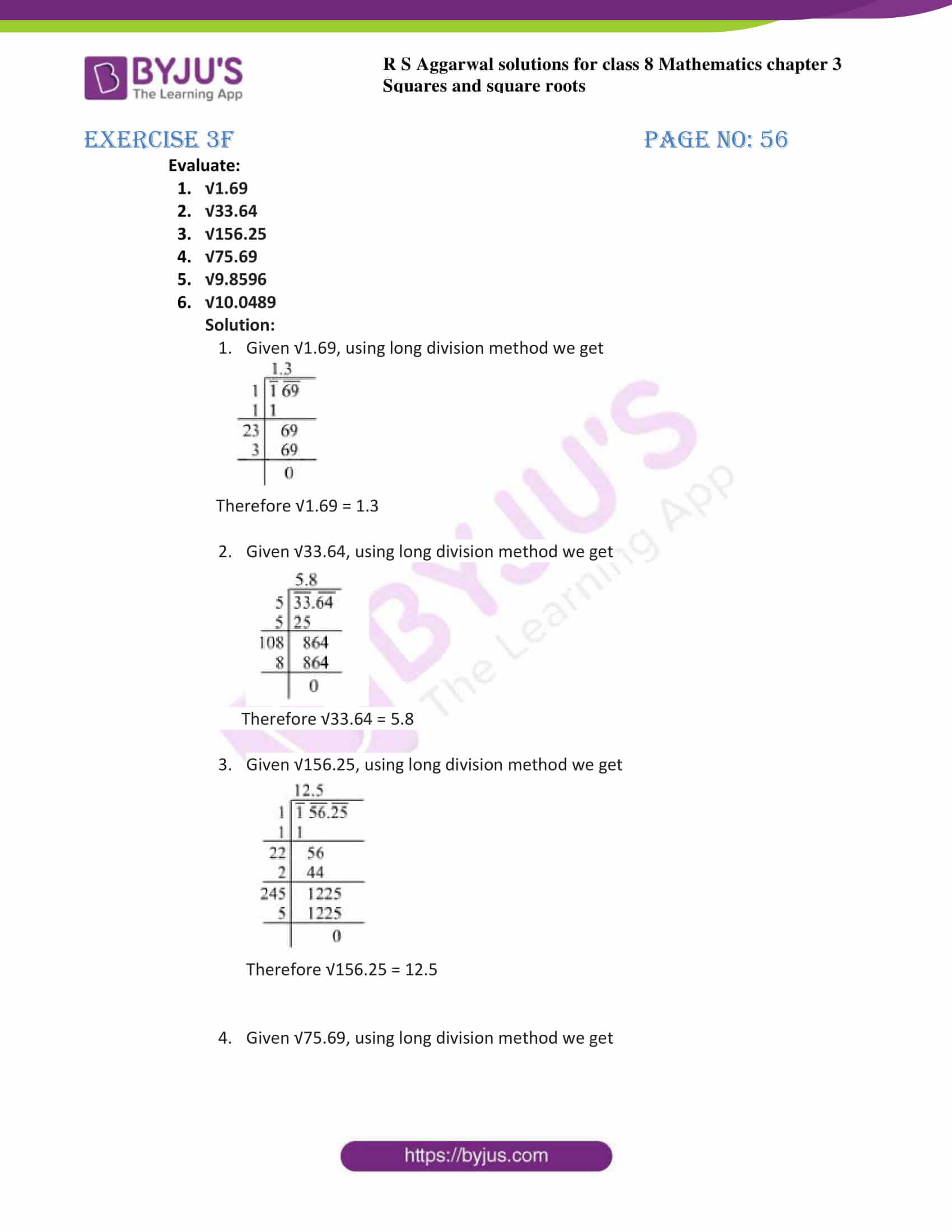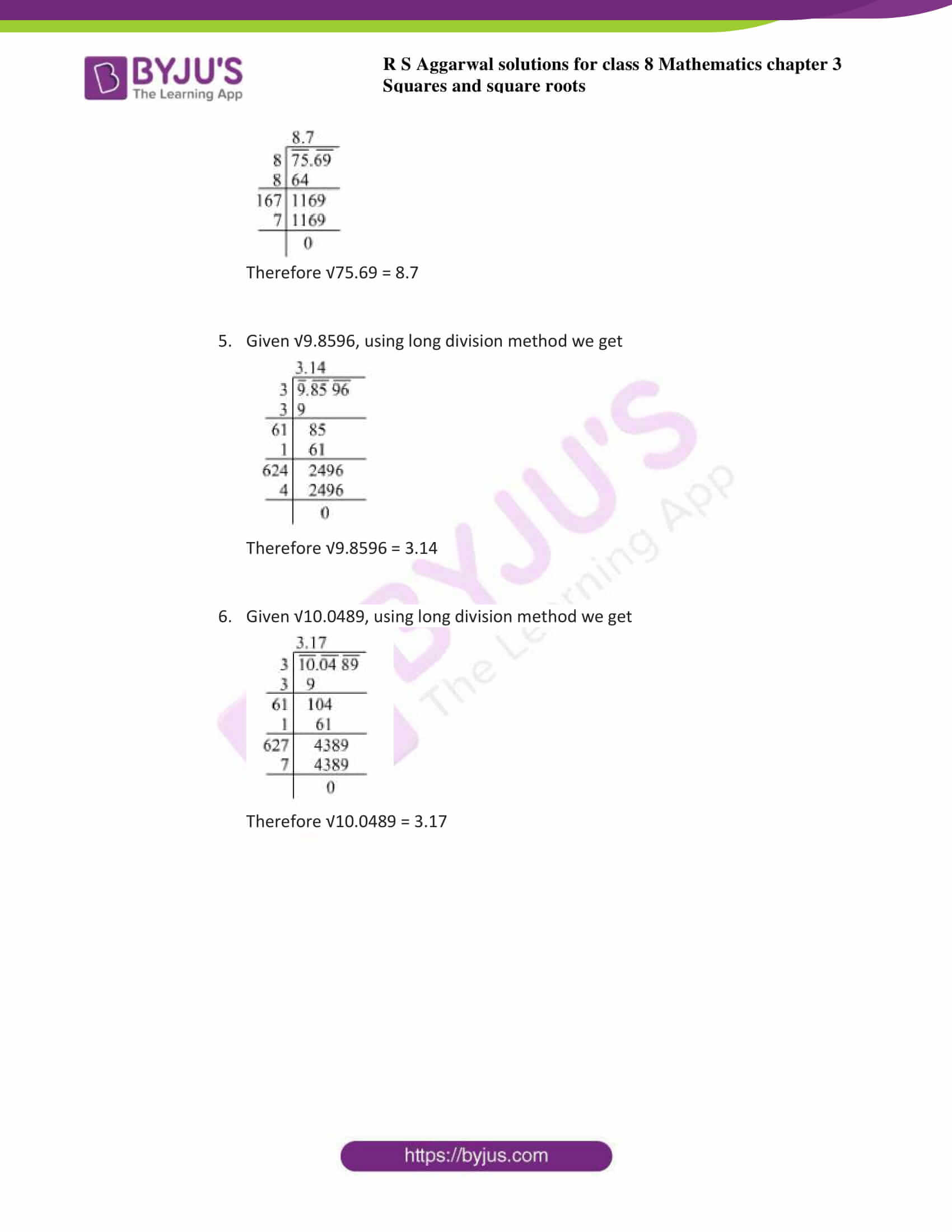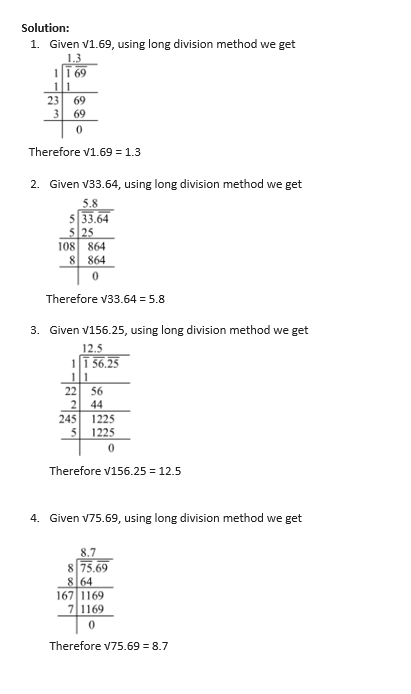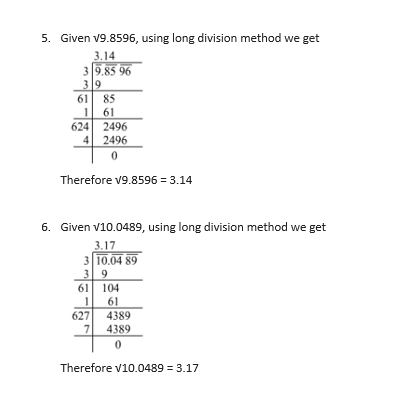# RS Aggarwal Solutions for Class 8 Chapter 3 - Square and Square Roots Exercise 3F

Students can access the link given below to RS Aggarwal Solutions for Class 8 exercise 3F. The questions present in the Exercise 3F have been solved by BYJU’S experts in Maths, and this will help students in solving Exercise 3F of class 8 without any problems. Exercise 3F of Class 8 helps students to understand the concept of finding the square root of a number in decimal form. By practising the RS Aggarwal Solutions for class 8, students will be able to grasp the concepts perfectly.

## Download PDF of RS Aggarwal Solutions for Class 8 Chapter 3 – Squares and Square Roots – Exercise 3F### Access answers to Maths RS Aggarwal Solutions for Class 8 Chapter 3 – Squares and Square Roots Exercise 3F

1. Evaluate:

1. √1.69
2. √33.64
3. √156.25
4. √75.69
5. √9.8596
6. √10.0489### Access other exercises of RS Aggarwal Solutions for Class 8 Chapter 3 – Squares and Square Roots

Exercise 3A Solutions 6 questions

Exercise 3B Solutions 13 questions

Exercise 3C Solutions 8 questions

Exercise 3D Solutions 18 questions

Exercise 3E Solutions 19 questions

Exercise 3G Solutions 10 questions

Exercise 3H Solutions 19 questions

## RS Aggarwal Solutions for Class 8 Maths Chapter 3 – Squares and Square Roots Exercise 3F

Exercise 3F of RS Aggarwal Solutions for Chapter 3, Squares and Square Roots deals with the basic concepts related to square roots. This exercise contains 12 questions based on finding a square root of a given decimal number. We can say that this exercise mainly deals with the methods to find square roots of decimals that the students have learned in this chapter. Some of the topics focused prior to exercise 3F include the Square roots of decimals

RS Aggarwal Solutions can help the students in practising and learning each and every concept as it provides solutions to all questions asked in the RS Aggarwal textbook. Those who aim to score high in Maths of class 8 are advised to practice all the questions present in RS Aggarwal as many times as possible. Very lengthy questions that require many steps can be easily remembered by the students by using RS Aggarwal Solutions.